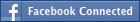• NEW! FREE Beat The GMAT QuizzesHundreds of Questions Highly Detailed Reporting Expert Explanations
• 7 CATs FREE!
If you earn 100 Forum Points

Engage in the Beat The GMAT forums to earn
100 points for $49 worth of Veritas practice GMATs FREEVERITAS PRACTICE GMAT EXAMS Earn 10 Points Per Post Earn 10 Points Per Thanks Earn 10 Points Per Upvote ## Standard deviation ##### This topic has 1 expert reply and 4 member replies A certain list of 100 data has an average of 6 and a standard deviation of d, where d is positive. Which of the following pairs of data, when added to the list, must result in a list 0f 102 data with standard deviation less than d? A. -6 and 0 B. 0 and 0 C. 0 and 6 D. 0 and 12 E. 6 and 6 OA E Can anyone explain this in detail? Legendary MemberJoined 13 Mar 2010 Posted: 576 messages Followed by: 1 members Upvotes: 97 Standard deviation shows the is difference between the mean and the values,more the values closer to mean,lesser will be standard deviation. here the adding two values will make the sd lower mean ,since mean is 6 deviation of values will be lesser if the list have more number of values equal to mean(variance 0) ans option E _________________ “If you don't know where you are going, any road will get you there.” Lewis Carroll Senior | Next Rank: 100 PostsJoined 15 Aug 2009 Posted: 85 messages Upvotes: 19 my approach: sd= rt ((avg-x)^2...)/n) to decrease the sd, we need to decrease the numerator and increase the denominator which is increased to 102 to decrease the numerator, we need to decrease the the number squared, so the closer the number is to the avg, the lower the square that occurs with choice e Newbie | Next Rank: 10 PostsJoined 16 May 2010 Posted: 7 messages Upvotes: 1 Test Date: 6/12/2010 Target GMAT Score: 760 GMAT Score: 740 liferocks wrote: Standard deviation shows the is difference between the mean and the values,more the values closer to mean,lesser will be standard deviation. here the adding two values will make the sd lower mean ,since mean is 6 deviation of values will be lesser if the list have more number of values equal to mean(variance 0) ans option E Agree with this answer. This is a question that tests subject knowledge instead of computational skill. You must know that standard deviation is the average distance between any given point in the set and the mean of the set. If you want a smaller standard deviation, you need numbers that are closer to the mean, on average, than the rest of the set. _________________ MGMAT1: 720 (Q47, V41) GMATPrep1: 750 (Q48, V44) GMATPrep2: 770 (Q50, V47) Master | Next Rank: 500 PostsJoined 29 Mar 2010 Posted: 214 messages Upvotes: 37 GMAT Score: 700Think about it like this; the only way you can increase the data set and decrease the standard deviation (the amount the data deviates from average) would be to add two numbers that equal the average, or are very close to the average. Thus, E is correct. ### GMAT/MBA Expert GMAT InstructorJoined 21 May 2010 Posted: 1053 messages Followed by: 98 members Upvotes: 335 I agree with the solutions advanced. NO math is Standard deviation roughly measures the average distance between the mean and every value. To minimize standard deviation, the values added must be as close to the mean as possible. In this case the mean is 6, so the new values that would minimize standard deviation are 6 and 6. The answer is E. Look for topic="Statistics" and difficulty="500-600" to find similar questions in the drill generator. This is GMATPrep question 1155 Good luck, -Patrick _________________ Ask me about tutoring. •FREE GMAT Exam Know how you'd score today for$0

Available with Beat the GMAT members only code

•5-Day Free Trial
5-day free, full-access trial TTP Quant

Available with Beat the GMAT members only code

•Free Practice Test & Review
How would you score if you took the GMAT

Available with Beat the GMAT members only code

•Magoosh
Study with Magoosh GMAT prep

Available with Beat the GMAT members only code

•1 Hour Free
BEAT THE GMAT EXCLUSIVE

Available with Beat the GMAT members only code

•5 Day FREE Trial
Study Smarter, Not Harder

Available with Beat the GMAT members only code

•Free Veritas GMAT Class
Experience Lesson 1 Live Free

Available with Beat the GMAT members only code

•Free Trial & Practice Exam
BEAT THE GMAT EXCLUSIVE

Available with Beat the GMAT members only code

•Get 300+ Practice Questions

Available with Beat the GMAT members only code

•Award-winning private GMAT tutoring
Register now and save up to \$200

Available with Beat the GMAT members only code

### Top First Responders*

1Ian Stewart 44 first replies
2Brent@GMATPrepNow 34 first replies
3Scott@TargetTestPrep 32 first replies
4Jay@ManhattanReview 31 first replies
5GMATGuruNY 18 first replies
* Only counts replies to topics started in last 30 days
See More Top Beat The GMAT Members

### Most Active Experts

1Scott@TargetTestPrep

Target Test Prep

139 posts
2Max@Math Revolution

Math Revolution

90 posts
3Ian Stewart

GMATiX Teacher

53 posts
4Brent@GMATPrepNow

GMAT Prep Now Teacher

51 posts
5Jay@ManhattanReview

Manhattan Review

31 posts
See More Top Beat The GMAT Experts